The center of mass of a pitched baseball or radius 2.42 cm moves at 23.3 m/s. The ball spins about an axis through its center of mass with a

Question

The center of mass of a pitched baseball or radius 2.42 cm moves at 23.3 m/s. The ball spins about an axis through its center of mass with an angular speed of 158 rad/s. Calculate the ratio of the rotational energy to the translational kinetic energy. Treat the ball as a uniform sphere.

in progress 0
6 months 2021-07-22T00:33:59+00:00 1 Answers 4 views 0

1. To solve the problem, it will be necessary to define the rotational and translational kinetic energy in order to determine the relationship between the two. Rotational energy is defined as,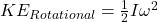Here,

I = Moment of Inertia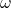= Angular velocity

Now the translational energy will be,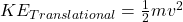Here,

m = Mass

v = Velocity

Therefore the relation between them will be,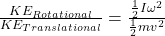Applying the moment of inertia of a sphere we have,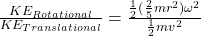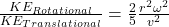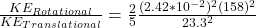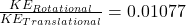Therefore the ratio will be 0.01077